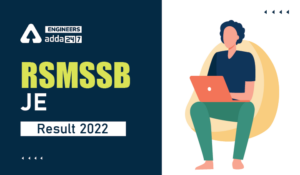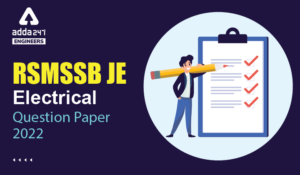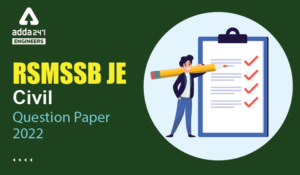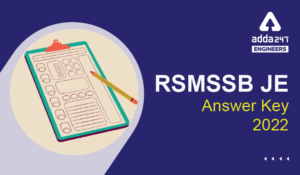Engineering Jobs   »   Civil Engineering Quiz

# RSMSSB-JE’21 CE: Daily Practices Quiz. 02-Sep-2021

As RSMSSB JE exam date 2021 is announced which is going to be held on 12th September 2021. As RSMSSB JE exam date 2021 is nearby so boost your preparation by attempt this Civil Engineering miscellaneous quiz for RSMSSB JE recruitment 2021.

Quiz: Civil Engineering
Exam: RSMSSB-JEn
Topic: Miscellaneous

Each question carries 1 mark
Negative marking: 1/3 mark
Time: 8 Minutes

Q1. A sewer is commonly designed to attain self-cleansing velocity at
(a) Sewer running half full
(b) Peak hourly rate of flow
(c) Minimum hourly rate of flow
(d) Average hourly rate of flow

Q2. Quick setting cement is produced by adding ______.
(a) Less amount of gypsum in very fine powdered form
(b) More amount of gypsum in very fine powdered form
(c) Aluminium sulphate in very fine powdered form
(d) Pozzolana in very fine powdered form

Q3. A summit curve is formed at the intersection of a 3% upgrade and a 5% downgrade what is the length of the summit curve in order to provide a stopping distance of 130 m.
(a) 271 m
(b) 298 m
(c) 307 m
(d) 340 m

Q4. Alkalinity in water is expressed as mg/l; in terms of: –
(a) Calcium carbonate
(b) Magnesium carbonate
(c) Sodium carbonate
(d) Sodium bi-carbonate

Q5. A test facility setup costs Rs 10,00,000 at the time of installation, and its scrap value is Rs 50,000 at the end of the useful life in 10 years. Adopting a straight-line method for computation of depreciation, estimate the book value of the facility.
(a) Rs 5,00,000
(b) Rs 5,25,000
(c) Rs 4,75,000
(d) Rs 5, 75, 000

Q6. 10 divisions of verniers scale are equal to 11 division of a main scale of each 0.1 mm. what is the least count of the verniers scale?
(a) 0.009
(b) 0.01
(c) 0.1
(d) 1.1

Solutions

S1. Ans.(c)
Sol. Self-cleansing velocity is such flow velocity as would be sufficient to flush out any deposited solids in the sewer. A sewer is commonly designed to attain the self-cleansing velocity at minimum hourly rate of flow.

S2. Ans.(c)
Sol. Quick Setting Cement as the name indicates, these types of cements are used where quick setting is needed. The quick setting cement is manufactured by adding a small percentage of aluminum sulphate and then it is finely grinded with cement. The Initial setting time of quick setting cement is 5 minutes and Final setting time is 30 minutes.

S3. Ans.(c)
Sol. Deviation angle = N = 3 + 5= 8% = 0.08
Assuming L > S
L = (NS^2)/4.4=(0.08×(130)^2)/4.4
= 307.2 m ≃ 307 m

S4. Ans.(a)
Sol. Alkalinity in water is expressed as mg/l in terms of calcium carbonate.
Major ions → CO_3^(-2),HCO_3^-,OH^-
Minor ions → HS^-,H_2 PO_4^-,HPO_4^(-2) etc.

S5. Ans.(b)
Sol. Given, Total cost (C) = 10,00,000 Rs
Scrap value (S) = 50,000 Rs
Design life (n) = 10 years
Annual depreciation by straight line Method→
D =(C-S)/n=(10,00,000-50,000)/10
▭(D=95000 Rs)
Depreciation value after – 5 years = 5 × 95000
= 4,75,000 Rs.
Book value after 5 years = 10,00,000 – 4,75,000
= 5,25,000

S6. Ans. (b)
Sol. Least count = (Smallest division of one main scale)/(no.of division on vernier scale ) = 0.1/10 = 0.01

Sharing is caring!

Thank You, Your details have been submitted we will get back to you.
•RSMSSB JE 2022 DV Schedule, Download RSM...
•RSMSSB JE Result 2022, OUT-Direct Link t...
•RSMSSB Computer Instructor Exam date 202...
•RSMSSB JE Electrical Question Paper 2022...
•RSMSSB JE Civil Question Paper 2022, Che...
•RSMSSB JE Answer Key 2022, Download RSMS...